# Noninverting op-amp circuit has simple gain formula

José Carrasco and Ausias Garrigós

EDN

Basic textbooks describe inverting and noninverting amplifiers based on operational amplifiers. These amplifiers have different gain equations. Whereas in the inverting configuration, the gain is the ratio of the feedback and input resistances, in the noninverting amplifier, the gain ratio has an added term. In some designs and for the sake of simplicity, it would be desirable to have a simple, proportional gain ratio (for gains above and below unity) for both inverting and noninverting amplifiers.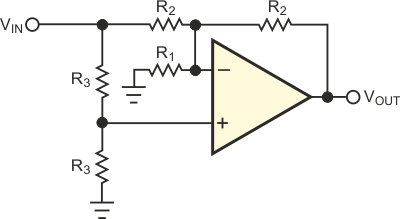Figure 1. This noninverting amplifier has a simple, proportional gain formula.

The noninverting amplifier in Figure 1 has a simple, proportional formula for the gain: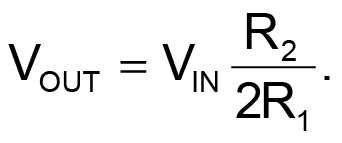This gain is proportional to a resistor ratio and can take any value. R3 has no influence on the gain. If you need a noninverting amplifier with a gain ratio identical to that of an inverting amplifier, the circuit in Figure 2 fills the bill. Its close-approximation gain formula is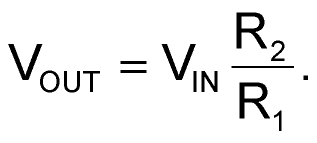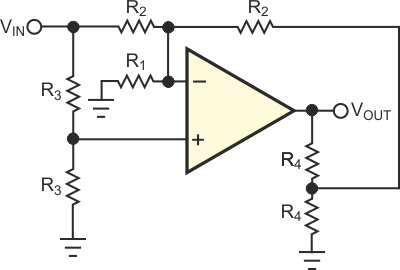Figure 2. This noninverting amplifier has a gain formula identical to that of an inverting amplifier.

However, in the circuit of Figure 2, some restrictions in the choice of R4 arise. The accuracy of the gain formula of the circuit depends on R4's being much lower in value than R1 or R2. Nevertheless, comparing the exact expression for the gain and the above approximation proves that R4 is 10 times lower in value than R1 or R2, whichever is smaller, and the gain error the approximation gives is less than 5%. For an exact computation, the error is equal to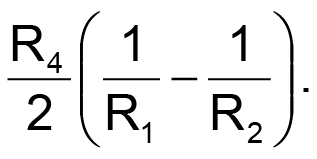For the selection of R4, you should take into account the fact that most op-amp ICs have maximum output currents of approximately 20 mA.

EDN